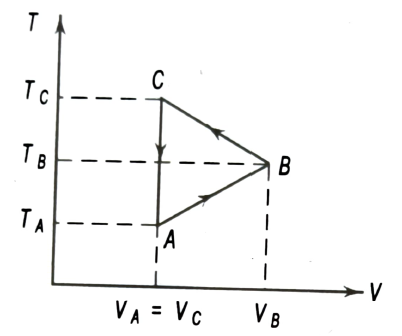Thermodynamic processes
Question

# Three moles of an ideal gas are taken through a cyclic process ABCA as shown on T-V diagram in Fig. The gas loses 2510 J of heat in the complete cycle. If . The work done by the gas during the process BC is….. (Take R = 8.3 JmolK-1)Moderate
Solution

## In the process AB, the volume V increases linearly with temperature T. hence AB is isobaric (constant pressure). Therefore, work done in this process is        $=\mathrm{nR}\left({\mathrm{T}}_{\mathrm{B}}-{\mathrm{T}}_{\mathrm{A}}\right)$      $=3×8.3×\left(200-100\right)=2490 \mathrm{J}$Process CA is isochoric (constant volume). Hence work done in this process WCA = 0. Since the whole process ABCA is cyclic, the change in internal energy in the complete cycle is zero, i.e., $∆\mathrm{U}=0$. Now, from the first law of the thermodynamics, (Given Q = -2510 J)$\mathrm{Q}=∆\mathrm{U}+\mathrm{W}=∆\mathrm{U}+{\mathrm{W}}_{\mathrm{AB}}+{\mathrm{W}}_{\mathrm{BC}}+{\mathrm{W}}_{\mathrm{CA}}$     $-2510=0+2490+{\mathrm{W}}_{\mathrm{BC}}+0$The negative sign shows that the work is done by the gas.

Get Instant Solutions